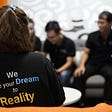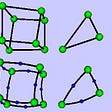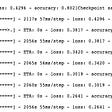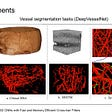# HOME CREDIT DEFAULT RISK

Loans have made life simpler for individuals. People take loans from banks for business and many other purposes to buy their dream home, dream car, and many others. Loan request is approved based on the loaners’ status, such as employment status, credit history, etc. However, the current existing evaluation system might not be appropriate to evaluate several loaners repayment ability, such as students or people without credit histories. And they usually end up taking loans from untrustworthy lenders who are likely to exploit borrowers by asking them to pay a high rate of interest.

Home Credit aims to include these subset of individuals by providing a safe and positive loan experience. It makes use of a variety of alternative data (e.g., including telco and transactional information) to predict their clients’ repayment abilities. Doing so will ensure that clients capable of repayment are not rejected and that loans are given with a principal, maturity, and repayment calendar that will empower their clients to be successful.

## Problem Statement:

Build a machine learning model that can predict the probability of the applicant’s capability to repay the loan.

1. Good AUC and Recall rate.
2. No low latency constraint.
3. Cost of misclassification is very high.

## Performance Metrics:

In this problem, the data is imbalanced. So we can’t use accuracy as an error metric. We can use Recall, Precision and AUC.

1. ROC-AUC Score: ROC is a probability curve and AUC represents the degree or measure of separability. It tells you to what extent the model is capable of distinguishing between classes. The higher the AUC, the better the model is at predicting 0s as 0s and 1s as 1s(model at distinguishing between the positive and negative classes).
2. Recall Score: It is the ratio of the True Positives predicted by the model and the total number of Actual Positives. It is also known as True Positive Rate.
3. Precision Score: It is the ratio of True Positives and the Total Positives predicted by the model.

4. Confusion Matrix :To get an overview of complete predictions. It tells about misclassifications for both the classes.

## Dataset Description:

There are 8 different tables of data:

1. application_{train|test}.csv : Static data for all applications. One row represents one loan in our data sample.
2. bureau.csv : All client’s previous credits provided by other financial institutions that were reported to Credit Bureau (for clients who have a loan in our sample).
3. bureau_balance.csv : Monthly balances of previous credits in the Credit Bureau.
4. POS_CASH_balance.csv : Monthly balance snapshots of previous POS (point of sales) and cash loans that the applicant had with Home Credit.
5. credit_card_balance.csv : Monthly balance snapshots of previous credit cards that the applicant has with Home Credit.
6. previous_application.csv : All previous applications for Home Credit loans of clients who have loans in our sample. There is one row for each previous application related to loans in our data sample.
7. installments_payments.csv : Repayment history for the previously disbursed credits in Home Credit related to the loans in our sample.
8. HomeCredit_columns_description.csv : This file contains descriptions for the columns in the various data files.

## Mapping the real-world problem to an ML problem:

It is a Supervised learning classification problem. Given the data of particular loan application, we should be able to predict whether the applicant is capable of repayment or not.

Since we have 2 classes in the target label, it is a Binary Classification Problem.

# Exploratory Data Analysis:

a. application_{train|test}.csv

## Distribution of Target Variable

Analysis:

• If the Target Variable: 0 = loan repaid,1 = loan not repaid.
• We can clearly see from the countplot that the number of customers who repaid the loan(0) are more than who did not repay the loan(1).Less than 50,000 of the total customers were not able to repay the loan and more than 2,50,000 of them repaid the loan.

## Distribution of Categorical Variables

NAME_CONTRACT_TYPE : Identification if loan is cash or revolving

Analysis

• There are two types of loan in our dataset = cash and revolving loan
• From 1st subplot we can see that most contract type of clients is Cash loans. Revolving loans are just a small fraction (9.5%) from the total number of loans.
• From 2nd subplot we can see that customers with Cash loans were not able to repay loan compared to Revolving loans.

CODE_GENDER : Gender of the client

Analysis

• From the Pie-Plot on the left side, we see that there are more Female Clients that have applied for loan as compared to Male Clients.
• However, if we look at the Percentage of Defaulters for each category, we see that it is the Males who tend to have Defaulted more than Females.

Distribution of Numerical features

DAYS_BIRTH : Client’s age in days at the time of application

Analysis:

• For easier interpretability, we have converted DAYS_BIRTH feature into AGE_YEARS by dividing it by 365.
• From first subplot, we can tell that most Loan applicants are around 40 years of age .
• From the second subplot, we can observe the highest peak is for defaulters, there are more number of applicants who did not repay the loan than the applicants who repaid the loan. Age group between (20–40) have difficulty in repaying the loan.
• As the age increases, we see age group (50–70) are more likely to repay the loan.
• There is visible separation between classes, so it’s a useful feature.

EXT_SOURCE_1,_2,_3 : Normalized score from external data source

Analysis:

• EXT_SOURCE_1 : The peak for Defaulters is higher at lower values of the variable, while for Non-Defaulters, it is higher at higher values of the variables.
• EXT_SOURCE_2 and EXT_SOURCE_3 : The peak for Defaulters and Non-Defaulters is higher at higher values of the variable.
• EXT_SOURCE_1, EXT_SOURCE_2, EXT_SOURCE_3 features are very useful, there is visible separation between two classes.

DAYS_EMPLOYED

Analysis:

• For easier interpretability, we have converted this feature into YEARS_EMPLOYED by dividing it by 365
• After plotting number of years employed, there are some clients in both target classes who are working for 1000 years which are outliers.
• After removing the value 365243 in number of days employed now the maximum number of years a person has worked is 50 years(3rd plot).
• Applicants who have less than 10 years of experience had difficulties in repaying the loan.

## AMT_INCOME_TOTAL

Analysis:

• AMT_INCOME_TOTAL is the income of the client.
• From the above plot, we can see that people with high income(>10,00,000) are likely to repay the loan.

# Feature Engineering and Data Preprocessing

application_train.csv :

1. Data Cleaning:

2. Deriving new features:

3. One hot encoding of categorical variables:

bureau.csv & bureau_balance.csv:

1. One hot coding of categorical features, created new features and performed numerical aggregations to roll up 27299925 rows in bureau_balance to 817395 rows at ‘SK_ID_BUREAU’ level.

2. Merged bureau with aggregated bureau balance and created manual features.

## previous_application.csv:

1. Data Cleaning: We found some erroneous values in DAYS columns(Relative to application date of current application), so we will replace them with NaN values.

2. One hot coding of categorical features, created new features and performed numerical aggregations to roll up 1670214 rows in Prev_app to 338857 rows at ‘SK_ID_BUREAU’ level.

## installments_payments:

1. Created Manual Features in installment_payments and performed numerical aggregations to roll up 1670214 rows in Prev_app to 338857 rows at ‘SK_ID_BUREAU’ level.

POS_CASH_balance.csv:

1. One hot coding of categorical features and performed numerical aggregations to roll up 10001358 rows to 337252 rows at ‘SK_ID_BUREAU’ level.
2. Grouped data based on NAME_CONTRACT_STATUS and then applied aggregations.

## credit_card_balance.csv:

1. One hot coding of categorical features and performed numerical and categorical aggregations to roll up 3840312 rows to 103558 rows at ‘SK_ID_BUREAU’ level.

Saving all preprocessed tables:

Merging tables:

Merging bureau_balance_agg, credit_card, prev_app, installments_payments and POS_CASH_balance. This merged table contains all previous data for a SK_ID_CURR(loan id).

## Handling Missing Values:

1. Replacing inf values with nan and deleting columns with greater than 75% missing values

2. Imputing missing values in train data with median values and scale using MinMaxScaler.

# Model tuning and selection:

I have tried Logistic Regression, Random Forest and LightGBM machine learning models.

# 1. Logistic Regression with hyperparameter tuning:

• alpha : hyperparameter.
• We will use SGDClassifier with log-loss, l2 penalty and class balancing.
`alpha                 = [10 ** x for x in range(-6, 3)]cv_score              = []train_score           = []cv_log_error_array    = []train_log_error_array = []for i in alpha:    print("for alpha =", i)    model1 = SGDClassifier(class_weight='balanced', alpha=i,      penalty='l2', loss='log', random_state=42)    model1.fit(X_train,y_train)    model1_CC = CalibratedClassifierCV(model1, method="sigmoid")    model1_CC.fit(X_train,y_train)    cv_predict = model1_CC.predict_proba(X_cv)    x_train_predict = model1_CC.predict_proba(X_train)    train_score.append(roc_auc_score (y_train, x_train_predict[:,1],  labels=model1.classes_))    cv_score.append(roc_auc_score(y_cv,cv_predict[:,1],  labels=model1.classes_))    cv_log_error_array.append(log_loss(y_cv,cv_predict, labels=model1.classes_, eps=1e-15))    train_log_error_array.append(log_loss(y_train, x_train_predict, labels=model1.classes_, eps=1e-15))    print("Train Log Loss :",log_loss(y_train, x_train_predict))    print("CV Log Loss :",log_loss(y_cv, cv_predict))`

ROC-AUC score:

## Threshold-Moving for Imbalanced Classification:

There are many techniques that may be used to address an imbalanced classification problem, such as resampling the training dataset and developing customized version of machine learning algorithms.

Nevertheless, perhaps the simplest approach to handle a severe class imbalance is to change the decision threshold.

`# calculate roc curvesfpr, tpr, thresholds = metrics.roc_curve(y_train,predicted_train[:,1] predicted_train[:,1])# get the best thresholdJ = tpr - fprix = np.argmax(J)best_thresh = thresholds[ix]def proba_to_class(threshold,proba):    return np.where(proba > threshold, 1, 0)predicted_train_new=proba_to_class(best_thresh,predicted_train[:,1])predicted_cv_new    = proba_to_class(best_thresh, predicted_cv[:,1])print("Train Data Results after moving threshold:")print('Best Threshold   =    %f' % (best_thresh))print("ROC-AUC Score=",roc_auc_score(y_train, predicted_train[:,1]))print("Precision Score =",precision_score(y_train, predicted_train_new))print("Recall Score =  ",recall_score(y_train, predicted_train_new))print("\nConfusion Matrix of Training data:\n")conf_mat = confusion_matrix(y_train, predicted_train_new)conf_mat = pd.DataFrame(conf_mat, columns = ['Predicted_0','Predicted_1'], index = ['Actual_0','Actual_1'])plt.figure(figsize = (7,6))plt.title('Confusion Matrix Heatmap')sns.heatmap(conf_mat, annot = True, fmt = 'g', linewidth = 0.5, annot_kws = {'size' : 15})plt.show()`

# 2. Random Forest with hyperparameter tuning:

• Here we will use Randomized Search technique for hyperparameter tuning.

Training Random Forest using best hyperparameters

Results after Threshold-Moving:

# 3. Light Gradient Boosting Mechanism:

• Light GBM is a gradient boosting framework that uses tree based learning algorithms.
• Light GBM is prefixed as ‘Light’ because of its high speed. Light GBM can handle the large size of data and takes lower memory to run.
• Here Bayesian Optimization is used for hyperparameter tuning.
`def lgbm_evaluation(num_leaves, max_depth, min_split_gain,                     min_child_weight,min_child_samples, subsample,                     colsample_bytree, reg_alpha, reg_lambda):    params = {'objective' : 'binary',              'boosting_type' : 'gbdt',              'learning_rate' : 0.005,              'n_estimators' : 10000,              'n_jobs' : -1,              'num_leaves' : int(round(num_leaves)),              'max_depth' : int(round(max_depth)),              'min_split_gain' : min_split_gain,              'min_child_weight' : min_child_weight,              'min_child_samples' : int(round(min_child_samples)),              'subsample': subsample,              'subsample_freq' : 1,              'colsample_bytree' : colsample_bytree,              'reg_alpha' : reg_alpha,              'reg_lambda' : reg_lambda,              'verbosity' : -1,              'seed' : 266    }    stratified_cv = StratifiedKFold(n_splits = 3, shuffle = True,                                      random_state = 33)    cv_preds = np.zeros(train_data.shape)        for train_indices, cv_indices in stratified_cv.split(train_data,                                      train_label):        x_tr = train_data.iloc[train_indices]        y_tr = train_label.iloc[train_indices]        x_cv = train_data.iloc[cv_indices]        y_cv = train_label.iloc[cv_indices]        lgbm_clf = lgb.LGBMClassifier(**params)        lgbm_clf.fit(x_tr, y_tr, eval_set= [(x_cv, y_cv)],                     eval_metric='auc', verbose = False,                      early_stopping_rounds=200)        cv_preds[cv_indices] = lgbm_clf.predict_proba(x_cv,                     num_iteration = lgbm_clf.best_iteration_)[:,1]    return roc_auc_score(train_label, cv_preds)bopt_lgbm = BayesianOptimization(lgbm_evaluation,             {'num_leaves' :  (25,50),             'max_depth' : (6,11),             'min_split_gain' : (0, 0.1),             'min_child_weight' : (5,80),             'min_child_samples' : (5,80),             'subsample' : (0.5,1),             'colsample_bytree' : (0.5,1),             'reg_alpha' : (0.001, 0.3),             'reg_lambda' : (0.001, 0.3)},              random_state = 4976)bayesian_optimization = bopt_lgbm.maximize(n_iter = 6, init_points = 4)`

The best ROC-AUC score is obtained at the 2nd iteration. Using the best values of a parameters of LGBM we do 10 fold cross-validation.

Results after Threshold-Moving:

# Result

Final comparison of models output:

# Deployment using Streamlit:

• After training the LightGBM (best model) with best hyper-parameters we stored the model in pickle file and deployed model on my local system along with Streamlit built around the final pipeline which takes SK_ID_CURR as input and returns the Predicted probability of default.

# Future Work

One can learn more about finance domain and add some more features to improve the AUC score. Also try to add some Deep learning and Stacked ensemble model Models to improve the kaggle score board.

--

--

--

## More from Ashwini

Love podcasts or audiobooks? Learn on the go with our new app.

## Machine learning project pipeline## TensorFlow on Mobile: Tutorial## How to Improve Your Machine Learning Predictions … with Confidence## Steps involved in finite element analysis.## Sentiment Analysis with BERT## 3D Cross-Hair Convolutional Neural Networks## Explaining YoloV4 a one stage detector## Trip to Grand Canyon## Scottie Barnes’ scoring## Southern Photos — Part 1## How to watch HBO Max on Roku